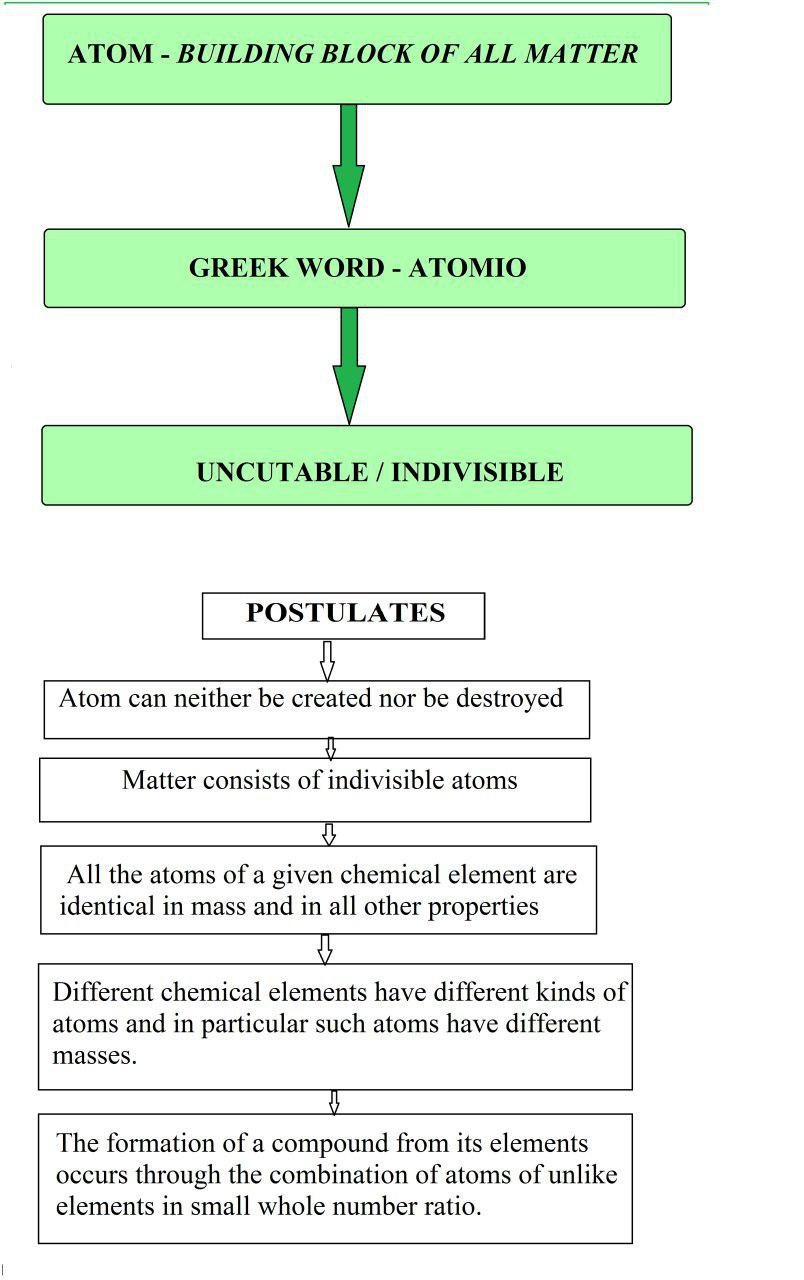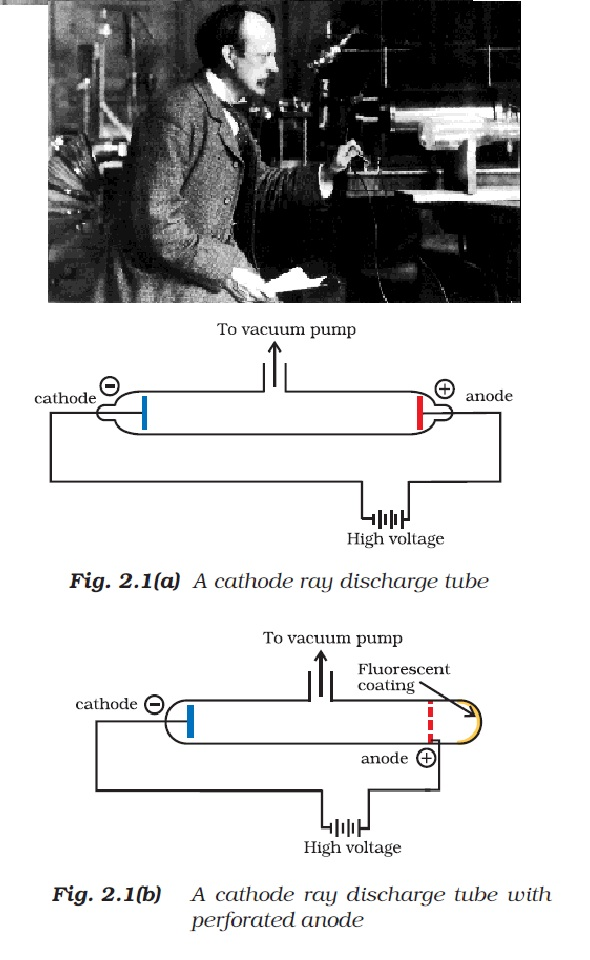Chemistry Atom, Dalton's Atomic Theory , Discovery of Sub-Atomic Particles , Charge to Mass Ratio of Electron , Charge on the Electron
Click for Only Video

### Topics to be covered :

This lecture covers following topics :

● Introduction to Atom
● Dalton's Atomic Theory
● Discovery of Sub-atomic Particles and their Characteristics.

● Charge to Mass Ratio of Electron .

● Charge on the Electron .

### Atom :

The word ‘atom’ has been derived from the Greek word ‘a-tomio’ which means ‘uncutable’ or ‘non-divisible’.

### Dalton’s Atomic Theory :John Dalton first proposed the atomic theory of matter in 1808 called Dalton’s Atomic Theory. This theory regarded the atom as the ultimate particle of matter.

But towards the end of the 19th and beginning of 20th century divided into sub-atomic particles, i.e., electrons, protons and neutrons. This concept was very different from that of Dalton.

Dalton’s atomic theory was able to explain :-

(a) the law of conservation of mass
(b) the law of constant composition and
(c) law of multiple proportion

However, it failed to explain the electric charges and attraction and repulsion between them.

### Discovery of Sub-Atomic Particles :Discovery of Electron :

Faraday began to study electrical discharge in partially evacuated tubes, known as cathode ray discharge tubes.
See fig2.1(a) and fig.2.1(b).

=> A cathode ray tube is made of glass containing two thin pieces of metal, called electrodes, sealed in it.

=> The electrical discharge through the gases could be observed only at very low pressures and at very high voltages. The pressure of different gases could be adjusted by evacuation.

=> When sufficiently high voltage is applied across the electrodes, current starts flowing through a stream of particles moving in the tube from the negative electrode (cathode) to the positive electrode (anode). These were called cathode rays or cathode ray particles.

=> The flow of current from cathode to anode was further checked by making a hole in the anode and coating the tube behind anode with phosphorescent material zinc sulphide. When these rays, after passing through anode, strike the zinc sulphide coating, a bright spot on the coating is developed(same thing happens in a television set)

The results of these experiments are summarised below :

(i) The cathode rays start from cathode and move towards the anode.

(ii) These rays are invisible but their behaviour can be observed with the help of certain kind of materials (fluorescent or phosphorescent) which glow when hit by them. Television picture tubes are cathode ray tubes.

(iii) In the absence of electrical or magnetic field, these rays travel in straight lines.

(iv) In the presence of electrical or magnetic field, the behaviour of cathode rays are similar to that expected from negatively charged particles, suggesting that the cathode rays consist of negatively charged particles, called electrons.

(v) The characteristics of cathode rays (electrons) do not depend upon the material of electrodes and the nature of the gas present in the cathode ray tube.

Thus, we can conclude that electrons are basic constituent of all the atoms.

### Charge to Mass Ratio of Electron :J.J. Thomson measured the ratio of electrical charge (e) to the mass of electron (me ) by using cathode ray tube and applying electrical and magnetic field perpendicular to each other as well as to the path of electrons (Fig. 2.2).

The amount of deviation of the particles from their path in the presence of electrical or magnetic field depends upon:

(i) The magnitude of the negative charge on the particle, greater the magnitude of the charge on the particle, greater is the
interaction with the electric or magnetic field and thus greater is the deflection.

(ii) the mass of the particle — lighter the particle, greater the deflection.

(iii) the strength of the electrical or magnetic field — greater the voltage across the electrodes, or the strength of the magnetic field, greater is the deflection.

When only electric field is applied, the electrons deviate from their path and hit the cathode ray tube at point A. Similarly when only magnetic field is applied, electron strikes the cathode ray tube at point C. By carefully balancing the electrical and magnetic field strength, it is possible to bring back the electron to the path followed as in the absence of electric or magnetic field and they hit the
screen at point B. By carrying out accurate measurements on the amount of deflections observed by the electrons on the electric field strength or magnetic field strength .

By carrying out accurate measurements on the amount of deflections observed by the electrons on the electric field strength or magnetic field strength, Thomson was able to determine the value of e//m_e as :

e//m_e = 1.758820 × 10^11 C kg^(-1)

Where m_e is the mass of the electron in kg and e is the magnitude of the charge on the electron in coulomb (C). Since electrons
are negatively charged, the charge on electron is –e .

### Charge on the Electron :R.A. Millikan (1868-1953) devised a method known as oil drop experiment (1906-14), to determine the charge on the electrons. He found that the charge on the electron to be – 1.6 × 10^(-19) C. The present accepted value of electrical charge is - 1.6022 × 10^(-19) C.

The mass of the electron (m_e) was determined as

e= e/(e//m_e) = 9.1094×10^(-31) kg